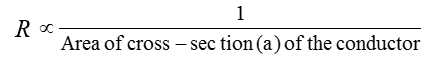Ex﻿

# 1. Electricity

#### Resistance Of Conductor

Resistance Of Conductor
The movement of electron gives rise to the flow of current through metals. The moving electrons collide with each other as well as with the positive ions present in the metallic conductor. These collisions tend to slow down the speed of the electrons and hence oppose the flow of electric current.
The property of a conductor by virtue of which it opposes the flow of electric current through it is called its resistance.
Resistance is denoted by the letter R.
The SI unit of resistance is ohm. The ohm is denoted by the Greek letter (Ω) called omega.
Resistance is a scalar quantity.
Factors on which resistance of conductor depends
Effect of the length on the resistance of a conductor The resistance of a conductor is directly proportional to the length. That is
Resistance of a conductor ∝ Length of the cond.
Effect of the area of cross-section on the resistance of a conductor
The resistance of a conductor is inversely proportional to its area of cross-section. That is,
Resistance of a conductor ;* If the area of cross-section of the conductor is doubled, its resistance gets halved.
Effect of temperature on the resistance of a conductor The resistance of all pure metals increases with a rise in temperature. The resistance of alloys increases very slightly with a rise in temperature. For metal when temperature increases resistance increases and for semiconductors when temperature increases resistance decreases.
Effect of the nature of material on the resistance of a conductor : Some materials have low resistance, whereas some others have much higher resistance. In general, an alloy has higher resistance than pure metals which from the alloy.
* Copper, silver, aluminium etc., have very low resistance.
* Nichrome, constantan etc., have higher resistance. Nichrome is used for making heating elements of heaters, toasters, electric iron etc.

If you want to give information about online courses to other students, then share it with more and more on Facebook, Twitter, Google Plus. The more the shares will be, the more students will benefit. The share buttons are given below for your convenience.
×

#### NTSE Physics (Class X)

• Electricity
• Magnetic Effect of Curent
• Light Reflection
• Light Refraction
• Human Eye & Colourful World
• Source of Energy
• Motion

#### NTSE Physics (Class IX)

• Force & laws of motion
• Work and Energy
• Gravitation
• Sound

SHOW CHAPTERS